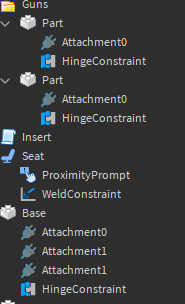# Turret wont rotate to mouse

im using hinges to rotate a turret towards a mouse. i gotten so close to doing it but it is off when turning aroundscript:

``````local goal = CFrame.lookAt(
rotateOrigin.Position,
mouse.Hit.Position
)

local y, x ,z = goal:ToOrientation()
print(x, y, z)
rotateHinge.TargetAngle = x * 360
``````

i also tried:

``````rotateHinge.TargetAngle = math.deg((mouse.Origin.Position - mouse.Hit.Position).Unit.X)
``````

this is done on a local script

1 Like

Try changing this line:

``````rotateHinge.TargetAngle = x * 360
``````

to this:

``````rotateHinge.TargetAngle = math.deg(x)
``````

CFrame:ToOrientation() returns the orientation in radians, so you have to use math.deg() to convert it back to degrees.

1 Like